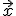• Have any questions?
• +91-9540-660064
• hahassignment@gmail.com

# Estimation Theory

Estimation theory is a branch of statistics and signal processing that deals with estimating the values of parameters based on measured/empirical data that has a random component. The parameters describe an underlying physical setting in such a way that the value of the parameters affects the distribution of the measured data.

The entire purpose of estimation theory is to arrive at an estimator, and preferably an implementable one that could actually be used. The estimator takes the measured data as input and produces an estimate of the parameters. It is also preferable to derive an estimator that exhibits optimality. Estimator optimality usually refers to achieving minimum average error over some class of estimators, for example, a minimum variance unbiased estimator. In this case, the class is the set of unbiased estimators, and the average error measure is variance (average squared error between the value of the estimate and the parameter). However, optimal estimators do not always exist.

These are the general steps to arrive at an estimator:

• In order to arrive at a desired estimator, it is first necessary to determine a probability distribution for the measured data, and the distribution's dependence on the unknown parameters of interest. Often, the probability distribution may be derived from physical models that explicitly show how the measured data depends on the parameters to be estimated, and how the data is corrupted by random errors or noise. In other cases, the probability distribution for the measured data is simply "assumed", for example, based on familiarity with the measured data and/or for analytical convenience.
• After deciding upon a probabilistic model, it is helpful to find the limitations placed upon an estimator. This limitation, for example, can be found through the Cramér–Rao bound.
• Next, an estimator needs to be developed or applied if an already known estimator is valid for the model. The estimator needs to be tested against the limitations to determine if it is an optimal estimator (if so, then no other estimator will perform better).
• Finally, experiments or simulations can be run using the estimator to test its performance.

Introduction to Interval Estimates:

Interval Estimate:

• Interval estimation is the process of calculate the interval for possible value of unknown parameter in the population.
• It is calculate in the use of sample data and contrast to the point estimation. It is different from the point estimation. It is the outcome of a statistical analysis.

The most common forms of interval estimations as follows:

• A frequents Method or Confidence interval
• A Bayesian method or credible intervals

The other common methods for interval estimations are

• Tolerance interval
• Prediction interval

And another one is known as the fiducial inference.

### Construction of Interval Estimates Parameter:

The normal form of interval estimate of the population parameter is,

• Point estimate of parameter and
• Plus or minus margin of error

Margin of error:

• The amount which is subtracted or added from  the point estimate  of the statistic and produce the parameter interval  estimate is known as the margin of error.
• The margin of error size depends on the following factors:
• Sampling distribution type of sample statistics.
• Area under sampling distribution percentage   that includes the researchers      decision.Usually we consider the confident level as 90%, 95%, 99%.
• The interval of each interval estimates are constructed in the region of the point estimate with its confident level.

### Construction of Interval Estimate for Population Mean

• Take the point estimate of μ  that is  the sample mean• Define  the mean distribution for the sample.When the  value of n is large we  have to use the central limit  theorem. And   is the normal distribution with the,

standard deviation sigmasigma/sqrt(n)

and mean μ.

• Choose the most common confident  level as 95%
• Find the margin of  error  which is related with the confidence level.
• The area  under the curve of  the sample means the normal distribution contains the 95%  of the interval from.

z= -1.96 to z= 1.96

• The interval estimate for 95 % is,- 1.96 (sigma/sqrt(n) ) tosigma/sqrt(n)

In case you face any problem or have any query please email us at :-info@homeworkassignmenthelp.com

### Submit us an Assignment:

For Demo Class Click here

Our tutors start working only after the payment is made, to ensure that we are doing work only for serious clients and also our solution meets the required standard.

### Getting homework help was never so easy you just need to follow following steps:

• Send us you Other Assignment or problem through email
• Specify the required format such as Word, Excel, Notepad, PDF
• Give us a deadline when you need the assignment completed along with the Time Zone.
(for example: EST, Australian GMT etc)
• Send documents related to your assignment which can help our tutors to provide a better work,
any example or format you want the solutions to be in.
• Our tutors will review the assignment sent by you and if all the required information is there we will
send you the price quoted by our tutor along with the time needed to solve the assignment
• You can pay us through paypal or credit card.
• After receiving the payment tutors start working on your assignment.
• Finally, we deliver the solutions and get a feedback from you regarding our work

In case you face any problem or have any query please email us at :- info@homeworkassignmenthelp.com